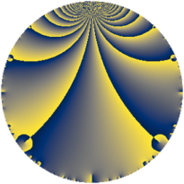Properties

 Label 4400.2.a.iLevel $4400$ Weight $2$ Character orbit 4400.a Self dual yes Analytic conductor $35.134$ Analytic rank $0$ Dimension $1$ CM no Inner twists $1$

Related objects

Newspace parameters

 Level: $$N$$ $$=$$ $$4400 = 2^{4} \cdot 5^{2} \cdot 11$$ Weight: $$k$$ $$=$$ $$2$$ Character orbit: $$[\chi]$$ $$=$$ 4400.a (trivial)

Newform invariants

 Self dual: yes Analytic conductor: $$35.1341768894$$ Analytic rank: $$0$$ Dimension: $$1$$ Coefficient field: $$\mathbb{Q}$$ Coefficient ring: $$\mathbb{Z}$$ Coefficient ring index: $$1$$ Twist minimal: no (minimal twist has level 11) Fricke sign: $$-1$$ Sato-Tate group: $\mathrm{SU}(2)$

$q$-expansion

 $$f(q)$$ $$=$$ $$q - q^{3} - 2 q^{7} - 2 q^{9} + O(q^{10})$$ $$q - q^{3} - 2 q^{7} - 2 q^{9} - q^{11} - 4 q^{13} + 2 q^{17} + 2 q^{21} - q^{23} + 5 q^{27} - 7 q^{31} + q^{33} - 3 q^{37} + 4 q^{39} - 8 q^{41} - 6 q^{43} + 8 q^{47} - 3 q^{49} - 2 q^{51} + 6 q^{53} - 5 q^{59} + 12 q^{61} + 4 q^{63} - 7 q^{67} + q^{69} + 3 q^{71} - 4 q^{73} + 2 q^{77} + 10 q^{79} + q^{81} - 6 q^{83} + 15 q^{89} + 8 q^{91} + 7 q^{93} + 7 q^{97} + 2 q^{99} + O(q^{100})$$

Embeddings

For each embedding $$\iota_m$$ of the coefficient field, the values $$\iota_m(a_n)$$ are shown below.

For more information on an embedded modular form you can click on its label.

Label $$\iota_m(\nu)$$ $$a_{2}$$ $$a_{3}$$ $$a_{4}$$ $$a_{5}$$ $$a_{6}$$ $$a_{7}$$ $$a_{8}$$ $$a_{9}$$ $$a_{10}$$
1.1
 0
0 −1.00000 0 0 0 −2.00000 0 −2.00000 0
 $$n$$: e.g. 2-40 or 990-1000 Significant digits: Format: Complex embeddings Normalized embeddings Satake parameters Satake angles

Atkin-Lehner signs

$$p$$ Sign
$$2$$ $$-1$$
$$5$$ $$1$$
$$11$$ $$1$$

Inner twists

This newform does not admit any (nontrivial) inner twists.

Twists

By twisting character orbit
Char Parity Ord Mult Type Twist Min Dim
1.a even 1 1 trivial 4400.2.a.i 1
4.b odd 2 1 275.2.a.b 1
5.b even 2 1 176.2.a.b 1
5.c odd 4 2 4400.2.b.h 2
12.b even 2 1 2475.2.a.a 1
15.d odd 2 1 1584.2.a.g 1
20.d odd 2 1 11.2.a.a 1
20.e even 4 2 275.2.b.a 2
35.c odd 2 1 8624.2.a.j 1
40.e odd 2 1 704.2.a.h 1
40.f even 2 1 704.2.a.c 1
44.c even 2 1 3025.2.a.a 1
55.d odd 2 1 1936.2.a.i 1
60.h even 2 1 99.2.a.d 1
60.l odd 4 2 2475.2.c.a 2
80.k odd 4 2 2816.2.c.j 2
80.q even 4 2 2816.2.c.f 2
120.i odd 2 1 6336.2.a.bu 1
120.m even 2 1 6336.2.a.br 1
140.c even 2 1 539.2.a.a 1
140.p odd 6 2 539.2.e.h 2
140.s even 6 2 539.2.e.g 2
180.n even 6 2 891.2.e.b 2
180.p odd 6 2 891.2.e.k 2
220.g even 2 1 121.2.a.d 1
220.n odd 10 4 121.2.c.e 4
220.o even 10 4 121.2.c.a 4
260.g odd 2 1 1859.2.a.b 1
340.d odd 2 1 3179.2.a.a 1
380.d even 2 1 3971.2.a.b 1
420.o odd 2 1 4851.2.a.t 1
440.c even 2 1 7744.2.a.x 1
440.o odd 2 1 7744.2.a.k 1
460.g even 2 1 5819.2.a.a 1
580.e odd 2 1 9251.2.a.d 1
660.g odd 2 1 1089.2.a.b 1
1540.b odd 2 1 5929.2.a.h 1

By twisted newform orbit
Twist Min Dim Char Parity Ord Mult Type
11.2.a.a 1 20.d odd 2 1
99.2.a.d 1 60.h even 2 1
121.2.a.d 1 220.g even 2 1
121.2.c.a 4 220.o even 10 4
121.2.c.e 4 220.n odd 10 4
176.2.a.b 1 5.b even 2 1
275.2.a.b 1 4.b odd 2 1
275.2.b.a 2 20.e even 4 2
539.2.a.a 1 140.c even 2 1
539.2.e.g 2 140.s even 6 2
539.2.e.h 2 140.p odd 6 2
704.2.a.c 1 40.f even 2 1
704.2.a.h 1 40.e odd 2 1
891.2.e.b 2 180.n even 6 2
891.2.e.k 2 180.p odd 6 2
1089.2.a.b 1 660.g odd 2 1
1584.2.a.g 1 15.d odd 2 1
1859.2.a.b 1 260.g odd 2 1
1936.2.a.i 1 55.d odd 2 1
2475.2.a.a 1 12.b even 2 1
2475.2.c.a 2 60.l odd 4 2
2816.2.c.f 2 80.q even 4 2
2816.2.c.j 2 80.k odd 4 2
3025.2.a.a 1 44.c even 2 1
3179.2.a.a 1 340.d odd 2 1
3971.2.a.b 1 380.d even 2 1
4400.2.a.i 1 1.a even 1 1 trivial
4400.2.b.h 2 5.c odd 4 2
4851.2.a.t 1 420.o odd 2 1
5819.2.a.a 1 460.g even 2 1
5929.2.a.h 1 1540.b odd 2 1
6336.2.a.br 1 120.m even 2 1
6336.2.a.bu 1 120.i odd 2 1
7744.2.a.k 1 440.o odd 2 1
7744.2.a.x 1 440.c even 2 1
8624.2.a.j 1 35.c odd 2 1
9251.2.a.d 1 580.e odd 2 1

Hecke kernels

This newform subspace can be constructed as the intersection of the kernels of the following linear operators acting on $$S_{2}^{\mathrm{new}}(\Gamma_0(4400))$$:

 $$T_{3} + 1$$ $$T_{7} + 2$$ $$T_{13} + 4$$

Hecke characteristic polynomials

$p$ $F_p(T)$
$2$ $$T$$
$3$ $$1 + T$$
$5$ $$T$$
$7$ $$2 + T$$
$11$ $$1 + T$$
$13$ $$4 + T$$
$17$ $$-2 + T$$
$19$ $$T$$
$23$ $$1 + T$$
$29$ $$T$$
$31$ $$7 + T$$
$37$ $$3 + T$$
$41$ $$8 + T$$
$43$ $$6 + T$$
$47$ $$-8 + T$$
$53$ $$-6 + T$$
$59$ $$5 + T$$
$61$ $$-12 + T$$
$67$ $$7 + T$$
$71$ $$-3 + T$$
$73$ $$4 + T$$
$79$ $$-10 + T$$
$83$ $$6 + T$$
$89$ $$-15 + T$$
$97$ $$-7 + T$$